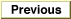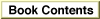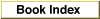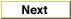# Legacy DocumentImportant: The information in this document is obsolete and should not be used for new development.

Inside Macintosh: QuickDraw GX Environment and Utilities /
Chapter 8 - QuickDraw GX Mathematics / Using QuickDraw GX Mathematics

## Analyzing the Bits in a Number

You can use the `FirstBit` function to determine the highest bit number that is set in a 32-bit number. The following examples demonstrate the use of this function with the parameter `x`:

If `x` is 1, the highest order bit that is set is bit number 0,
so `FirstBit(1)` = 0, as shown below.

`FirstBit(0000000000000000000000000000001) = 0x0000`

If `x` is 2, the highest order bit that is set is bit number 1, so `FirstBit(2)` = 1, as shown below.

`FirstBit(0000000000000000000000000000010) = 0x0001`

If `x` is 3, the highest order bit that is set is bit 1, so `FirstBit(3)` = 1, as shown below.

`FirstBit(0000000000000000000000000000011) = 0x0001`

If no bits in the number are set, `FirstBit` returns a value of -1.

You can also use `FirstBit` to find the last (= lowest-order) bit that is set in a number. Listing 8-9 is an example of such a function.

Listing 8-9 Determining the lowest bit of a number

```short LastBit(unsigned long x)
{
if (x == 0)
return 32;
return FirstBit(x & -x);
}
```
The `FirstBit` function is described on page 8-62.